# 问题1：梯度爆炸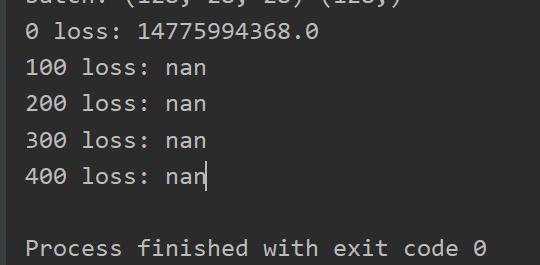``````w1 = tf.Variable(tf.random.truncated_normal([784,256], stddev=0.01))
b1 = tf.Variable(tf.zeros())
w2 = tf.Variable(tf.random.truncated_normal([256,128], stddev=0.01))
b2 = tf.Variable(tf.zeros())
w3 = tf.Variable(tf.random.truncated_normal([128,10], stddev=0.01))
b3 = tf.Variable(tf.zeros())
``````

# 问题2：数据没有跟踪到

``````with tf.GradientTape() as tape:
``````

# 补充内容-数据类型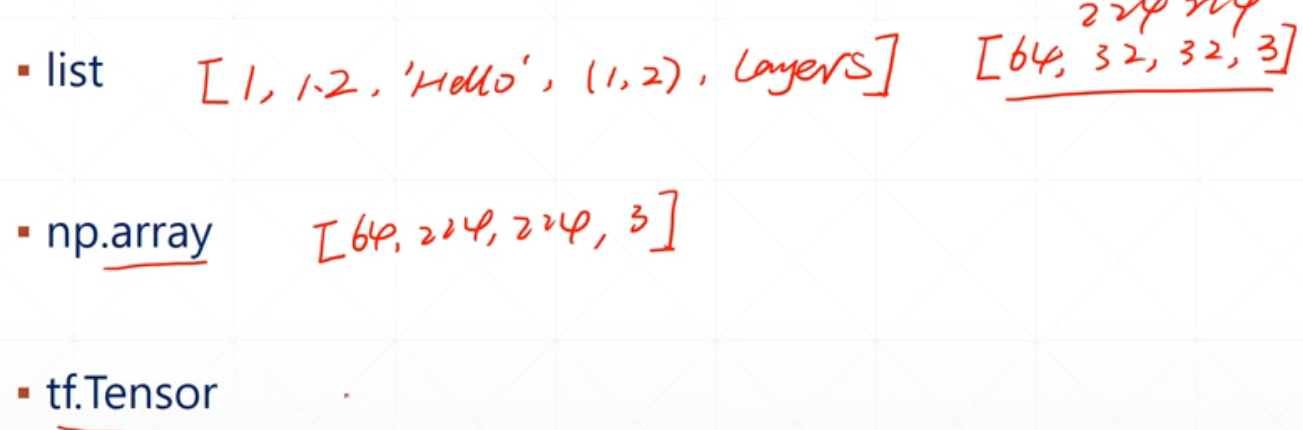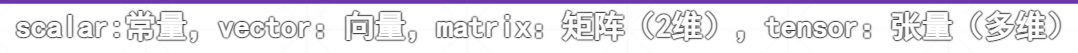• ndim，shape，numpy()，device，cpu/gpu
• 数据转换：cast指定dtype
• tensor包了一下tf.Variable就自动具有了求导的特性，也还是一个tensor
• Epoch：这一千张图片全部训练完成一次即为一个Epoch。
Step：1000/10 即为step，表示要更新多少次梯度。
BatchSize：这里的10 即为batchsize。
• 合并数据
concat：维度可以不同[4,35,8],[2,35,8]
stack：维度相同[4,35,8],[2,35,8]
• 切割数据
unstack：【2，4，35，8】假如在8这个维度打散，就得到了8个【2，4，35】
split：[【2，4，35，8】指定num_or_size_splites=2，则8/2=4就得到了两个【2，4，35，4】
• 数据统计
• 向量范数
二范数：tf.norm()=tf.sqrt(tf.reduce_sum(tf.square()))
一范数：指定ord=1
• 张量排序
sort排序，argsort排序前的位置（0开始）
top_k：最大的前几个
• 填充与复制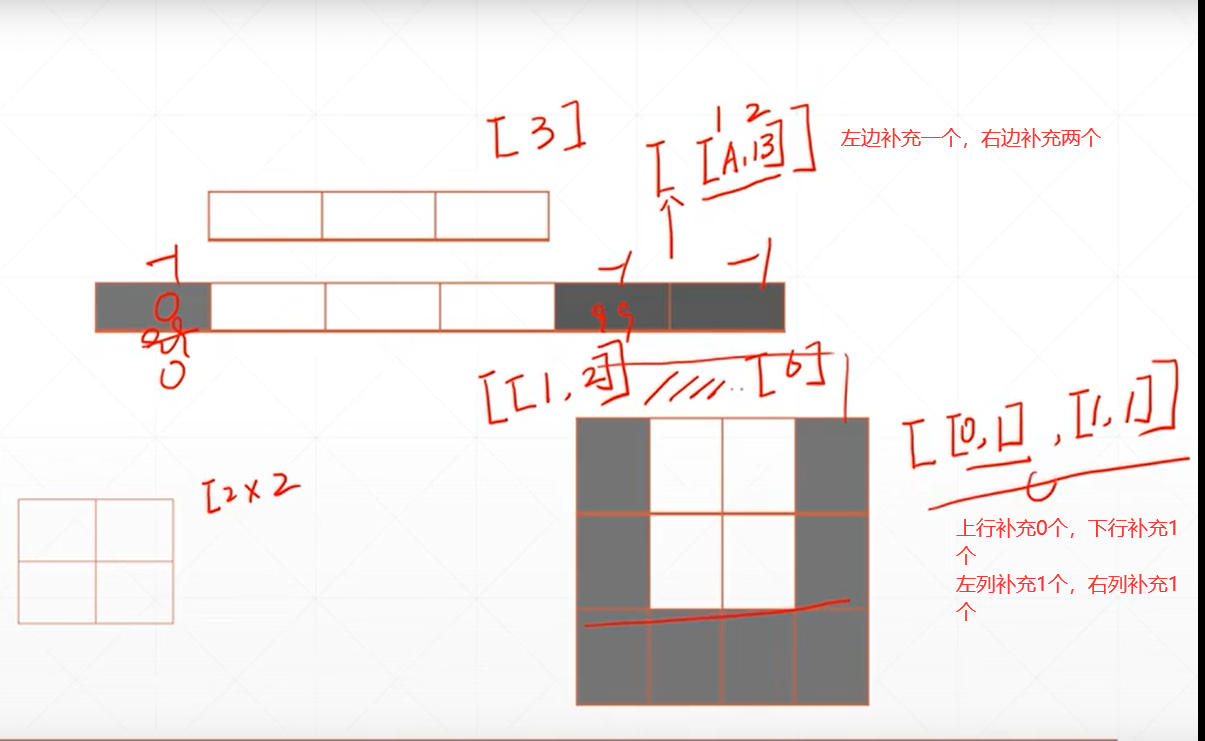tile：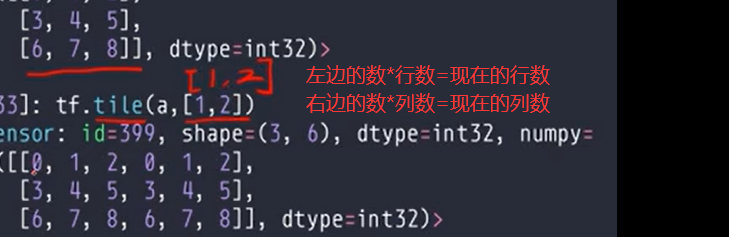• 张量限幅
exploding：值太大了。vanishing：值太小了，loss不动。
• tf.clip_by_global_norm：等比例的裁剪
• 高阶操作
• Where
• scatter_nd：有目的性的更新，在全0的底板上更新
• meshgrid：

Original: https://blog.csdn.net/qq_43634388/article/details/122849124
Author: 姬如
Title: TF学习day1